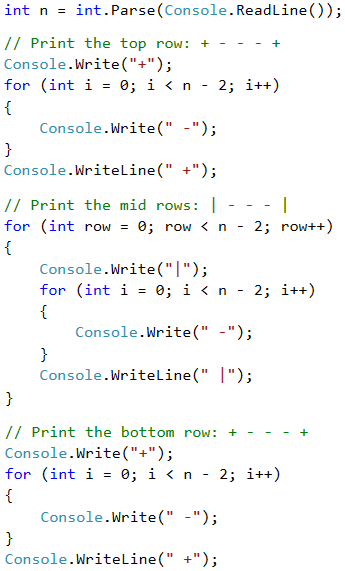# Example: Square Frame

Write a program that takes a positive integer n and draws on the console a square frame with a size of n * n.

Input Output Input Output
3 + - +
| - |
+ - +
4 + - - +
| - - |
| - - |
+ - - +
Input Output Input Output
5 + - - - +
| - - - |
| - - - |
| - - - |
+ - - - +
6 + - - - - +
| - - - - |
| - - - - |
| - - - - |
| - - - - |
+ - - - - +

## Video: Square Frame

Watch this video lesson to learn how to print a square frame on the console using nested loops: https://youtu.be/LS2uqvggfSA.

## Hints and Guidelines

We can solve the problem in the following way:

• We read from the console the number n.
• We print the upper part: first a + sign, then n-2 times - and in the end a + sign.
• We print the middle part: we print n-2 rows, as we first print a | sign, then n-2 times - and in the end again a | sign. We can do this with nested loops.
• We print the lower part: first a + sign, then n-2 times - and in the end a + sign.

Here is an example implementation of the above idea with nested loops: